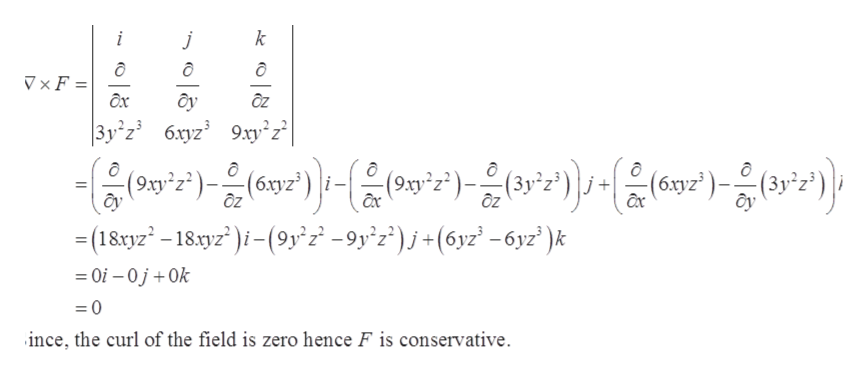# Determine whether or not the vector field is conservative. If it is conservative, find a function f such that F = ∇f. (If the vector field is not conservative, enter DNE.)F(x, y, z) = 3y2z3 i + 6xyz3 j + 9xy2z2 kf(x, y, z) =

Question
67 views

Determine whether or not the vector field is conservative. If it is conservative, find a function f such that F = ∇f. (If the vector field is not conservative, enter DNE.)

F(x, y, z) = 3y2z3 i + 6xyz3 j + 9xy2z2 k

f(xyz) =

check_circle

Step 1

To determine if the given field is conservative and find the potential function f(x,y,z).

Step 2

Given:

Step 3

Compute the curl of the vector field to det...help_outlineImage TranscriptioncloseV ×F = ôy ÔZ 3y°z 6.xyz 9.xy z| (6xyz³ ôy = (18xyz – 18.tyz" )i-(9y°z² -9y°z°)j+(6yz² -6yz' )k %3D = Oi – 0 j + Ok ince, the curl of the field is zero hence F is conservative. fullscreen

### Want to see the full answer?

See Solution

#### Want to see this answer and more?

Solutions are written by subject experts who are available 24/7. Questions are typically answered within 1 hour.*

See Solution
*Response times may vary by subject and question.
Tagged in

### Derivative